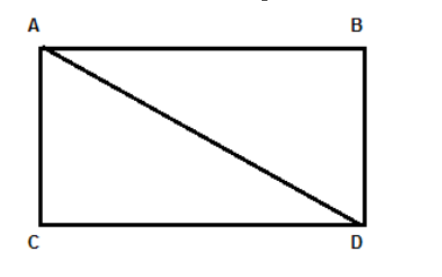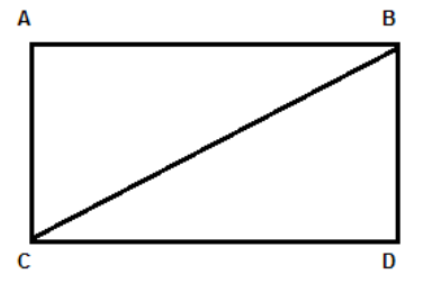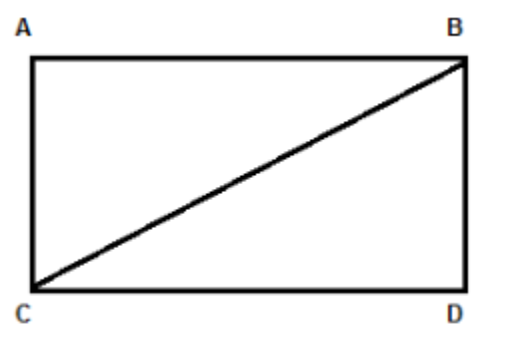Courses
Courses for Kids
Free study material
Free LIVE classes
MoreLIVE
Join Vedantu’s FREE Mastercalss

# Say true or false.The opposite sides of a rectangle are equal in length.(A) True(B) FalseVerified
361.8k+ views
Hint: Divide the rectangle into two parts diagonally. Then use Pythagoras theorem on the two triangles which are obtained after dividing diagonally. Then use the result to prove the lengths of the rectangle.

First let us consider a rectangle ABCD with AD as its diagonal, as shown below:Now the diagonal divides the rectangle into two equal parts as shown in the figure above.
Now as we know the sides of the rectangle are perpendicular to each other. So the two triangles obtained after dividing the rectangle diagonally will be right angled triangles.
Now consider the right angled triangle ABD. By Pythagoras theorem we have the sum of square of two sides is equal to the square of the hypotenuse, that is,
$A{{D}^{2}}=A{{B}^{2}}+B{{D}^{2}}...........(i)$
Similarly, consider the right angled triangle ACD. By Pythagoras theorem we have the sum of square of two sides is equal to the square of the hypotenuse, that is,
$A{{D}^{2}}=A{{C}^{2}}+C{{D}^{2}}...........(ii)$
Now as we can observe in equation (i) and (ii), the left hand side is equal. So equating these two equations, we get
$A{{B}^{2}}+B{{D}^{2}}=A{{C}^{2}}+C{{D}^{2}}$
$\Rightarrow A{{B}^{2}}=A{{C}^{2}}+C{{D}^{2}}-B{{D}^{2}}........(iii)$
Now let us divide the rectangle ABCD using the other diagonal, i.e., BC, as shown below:Now consider the right angled triangle ABC. By Pythagoras theorem we have the sum of square of two sides is equal to the square of the hypotenuse, that is,
$B{{C}^{2}}=A{{B}^{2}}+A{{C}^{2}}...........(iv)$
Similarly, consider the right angled triangle BCD. By Pythagoras theorem we have,
$B{{C}^{2}}=B{{D}^{2}}+C{{D}^{2}}...........(v)$
Now as we can observe in equation (iv) and (v), the left hand side is equal. So equating these two equations, we get
$A{{B}^{2}}+A{{C}^{2}}=B{{D}^{2}}+C{{D}^{2}}$
$\Rightarrow A{{B}^{2}}=B{{D}^{2}}+C{{D}^{2}}-A{{C}^{2}}........(vi)$
Now equating equation (iii) and (vi), we get
$A{{C}^{2}}+C{{D}^{2}}-B{{D}^{2}}=B{{D}^{2}}+C{{D}^{2}}-A{{C}^{2}}$
Cancelling like terms, we get
$A{{C}^{2}}-B{{D}^{2}}=B{{D}^{2}}-A{{C}^{2}}$
$\Rightarrow A{{C}^{2}}+A{{C}^{2}}=B{{D}^{2}}+B{{D}^{2}}$
$\Rightarrow 2A{{C}^{2}}=2B{{D}^{2}}$
$\Rightarrow A{{C}^{2}}=B{{D}^{2}}$
Taking square root on both sides, we get
AC = BD……..(vii)
Substituting this value in equation (iii), we get
$A{{B}^{2}}=A{{C}^{2}}+C{{D}^{2}}-A{{C}^{2}}$
$\Rightarrow A{{B}^{2}}=C{{D}^{2}}$
Taking square root on both sides, we get
$AB = CD$
Hence the opposite sides of a rectangle are equal in length. Therefore, the given statement is TRUE.
Therefore the correct answer is option (A).

Note: Another approach is considering diagonals of rectangle are equal. So, AD = BC.Now consider the right angled triangle ABC and ABD and apply the Pythagoras theorem. Here these two triangles are similar. So, they will give exact answer which shows the opposite sides are equal in length.
Last updated date: 22nd Sep 2023
Total views: 361.8k
Views today: 7.61k